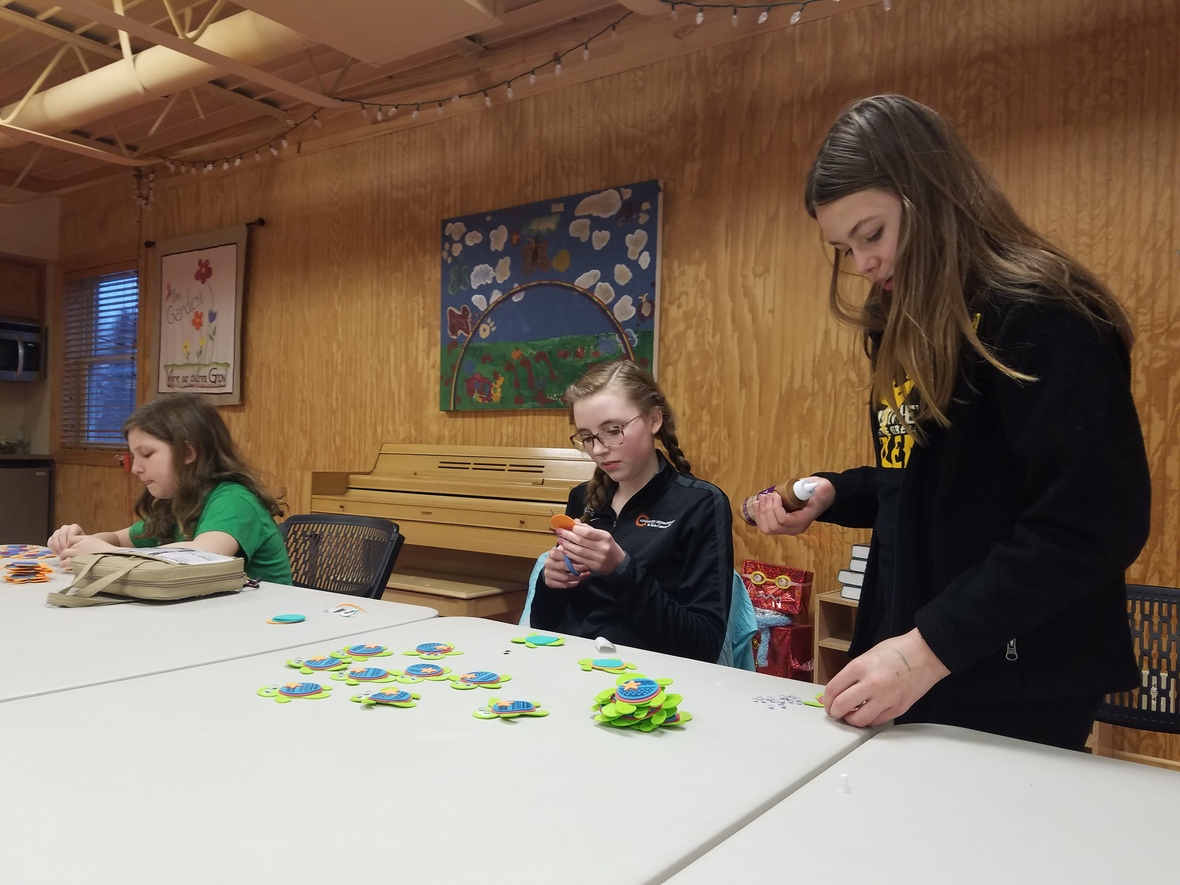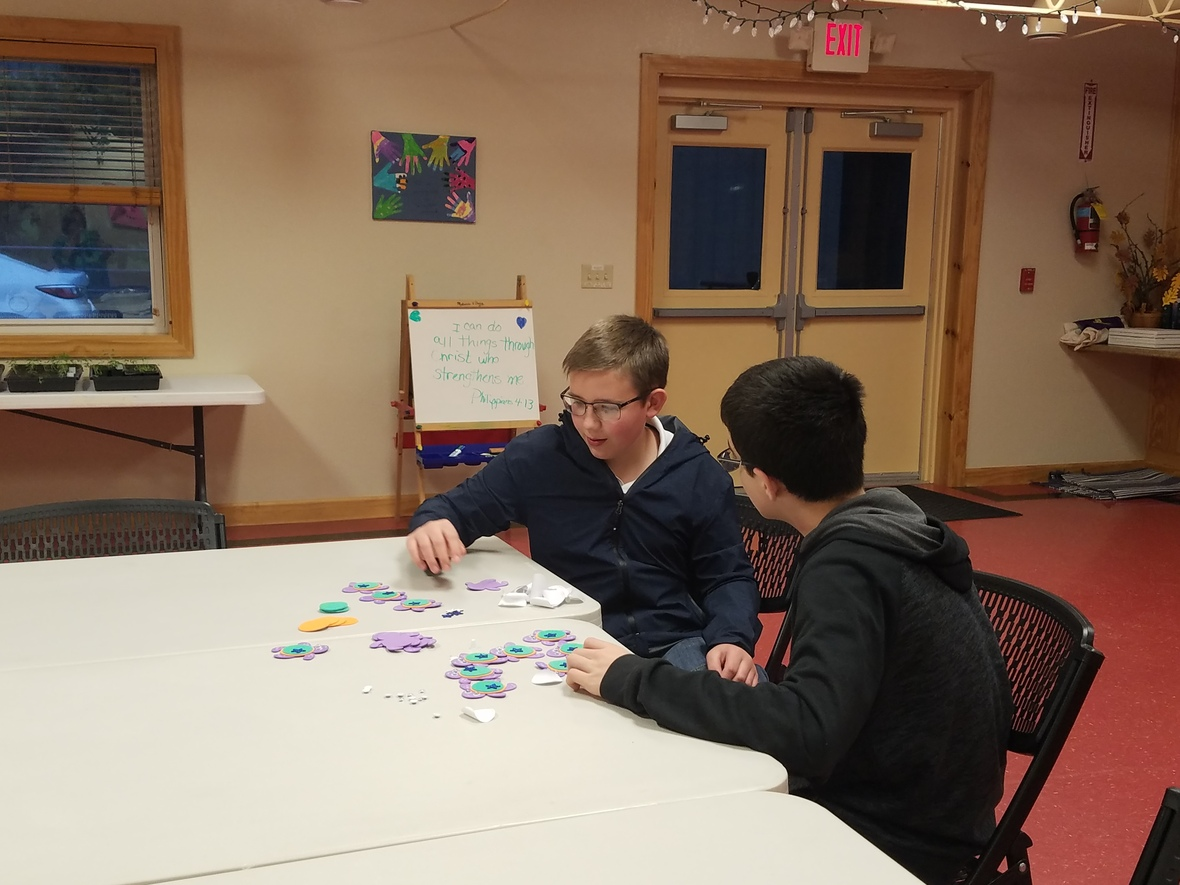# Sunday's Service - April 22, 2018

 /* styles */ We welcome back Adam Shank this week! Featured scripture verses are: 1 Corinthians 6:9-20 for his message: But You Were Washed! Our service this week will include the Baptism of Abigail McMaster. Join us this Sunday for Worship!
 table.module-4{width:0%;padding:0}table div table+table+table+table+table div table{width:0%;float:none;margin-left:auto;margin-right:auto;padding:0}table div table+table+table+table+table div table a{border:0 none;text-decoration:none}table div table+table+table+table+table div table img{width:100%!important;border:0 none;text-decoration:none}table div table+table+table+table+table div table td{width:100%;padding:0}/* styles */

# CG NEWS & EVENTS

## Garden News

Garden kids will have an abbreviated lesson this week, led by Jen Lynch. The group will return to participate in the Baptism celebration.

## Youth Group News

Last Sunday we talked about the disciples' experience as they witnessed the Transfiguration of Jesus. We also worked on a service project - making turtles to be distributed to the residents of Whispering Pines on Earth Day. All are welcome to attend the tree planting ceremony at Whispering Pines on Sunday at 2pm. This week we will focus on a lesson that is perfect for our location and this season of spring, called "Soul Gardening." (Snack: Audrey & Nathan Shannon)## Talent & Hobby Survey

Have YOU filled out a survey yet? There is still time! Check your email or visit the desk and let us know more about you. We would love to include your interests during events throughout the year! (The responses so far have been great - THANKS! More would be awesome!)

## Raised Beds Available!

Would you like to tend your own patch of the earth? Want to grow flowers to brighten your kitchen table or veggies to help you eat healthy this summer? Sign up for one of the raised beds that are in front of the church and you can be a gardener too! Let Jen Lynch or Rachel Vignon know if you are interested.

Pat Shannon leads a class on the book of Matthew on Sunday mornings from 9:30-10:30am.

We meet weekly on Wednesdays at 9am. Next week we begin the book Dwelling in God's Peace, from the Jesus Calling Bible Study Series by Sarah Young. You are welcome to join anytime, with or without the book! Little ones can come, too - they can play in the Sprouts Room while we meet in the Cafe. (Next week we may have a delayed start of 10am.)

## Friday Evening Bible Study

Bible study is held on Friday evenings at 6pm at the Shannon's house. Join in anytime!

# UPCOMING AT CG

## Abundance Orchard VBS

Do you have the week of July 9-13 marked on your calendar as VBS Week, from 10-12:30 each day? We are starting to get excited! Music Director Jess Deeter is already planning songs. Bible Adventure leader Jen Shannon is choosing our stories, so that we have a mix of Old and New Testament. We cannot wait for our time in the orchard with all of you!

## Outreach Potluck Luncheon

Celebrate the seasons after Worship on May 20th with a "Springing Into Summer!" potluck and ice cream sundae bar. Sign up sheet will be up soon.

 table div table+table+table+table+table+table+table+table+table+table+table+table+table+table+table+table+table+table div table{width:100%;padding:0}table div table+table+table+table+table+table+table+table+table+table+table+table+table+table+table+table+table+table div table img{width:96.23%;padding:0;float:none}table div table+table+table+table+table+table+table+table+table+table+table+table+table+table+table+table+table+table div table td{width:100%;padding:0 1.88% 18px}/* styles *//* styles */

# NEWS & EVENTS

 table div table+table+table+table+table+table+table+table+table+table+table+table+table+table+table+table+table+table+table+table+table+table div table,table.module-21{width:3.77%;float:right;padding:0}table div table+table+table+table+table+table+table+table+table+table+table+table+table+table+table+table+table+table+table+table+table+table div table a{border:0 none;text-decoration:none}table div table+table+table+table+table+table+table+table+table+table+table+table+table+table+table+table+table+table+table+table+table+table div table img{width:100%!important;border:0 none;text-decoration:none}table div table+table+table+table+table+table+table+table+table+table+table+table+table+table+table+table+table+table+table+table+table+table div table td{width:100%;padding:0 0 20px 20px}/* styles */ Tree Planting ~ This Sunday at 2:00 Goodness Grows will be out in the community this weekend, at Whispering Pines Village. We have recently joined forces with this facility and are planning ways we can interact with its residents. This special Earth Day observance is, hopefully, the first of many events we can do together! We will be there to take part in a tree planting ceremony and a flag raising. The American Legion will be retiring the old flag and putting up a new one. You are invited to join in and help us make community connections!
 table div table+table+table+table+table+table+table+table+table+table+table+table+table+table+table+table+table+table+table+table+table+table+table div table,table.module-22{width:3.77%;float:left;padding:0}table div table+table+table+table+table+table+table+table+table+table+table+table+table+table+table+table+table+table+table+table+table+table+table div table a{border:0 none;text-decoration:none}table div table+table+table+table+table+table+table+table+table+table+table+table+table+table+table+table+table+table+table+table+table+table+table div table img{width:100%!important;border:0 none;text-decoration:none}table div table+table+table+table+table+table+table+table+table+table+table+table+table+table+table+table+table+table+table+table+table+table+table div table td{width:100%;padding:0 20px 20px 0}/* styles */ Spring Plant & Flower Sale! May 9th & 10th from 9:00 to 2:00 May 11th from 9:00 to 7:00 * May 12th from 9:00 to 1:00 Have you seen what's growing in our greenhouse? Check it out and get ready to shop at our sale next month! Flowers for your yard, your sweetheart, a special teacher, or your mom. It's that time of year to spread flowers everywhere!
 table div table+table+table+table+table+table+table+table+table+table+table+table+table+table+table+table+table+table+table+table+table+table+table+table div table,table.module-23{width:3.77%;float:right;padding:0}table div table+table+table+table+table+table+table+table+table+table+table+table+table+table+table+table+table+table+table+table+table+table+table+table div table a{border:0 none;text-decoration:none}table div table+table+table+table+table+table+table+table+table+table+table+table+table+table+table+table+table+table+table+table+table+table+table+table div table img{width:100%!important;border:0 none;text-decoration:none}table div table+table+table+table+table+table+table+table+table+table+table+table+table+table+table+table+table+table+table+table+table+table+table+table div table td{width:100%;padding:0 0 20px 20px}/* styles */ Our clients made owl crafts this week, to go along with the Youth Group turtles. These will be given out at the Earth Day celebration on Sunday.
 table.module-24{width:0%;padding:0}table div table+table+table+table+table+table+table+table+table+table+table+table+table+table+table+table+table+table+table+table+table+table+table+table+table div table{width:0%;float:none;margin-left:auto;margin-right:auto;padding:0}table div table+table+table+table+table+table+table+table+table+table+table+table+table+table+table+table+table+table+table+table+table+table+table+table+table div table a{border:0 none;text-decoration:none}table div table+table+table+table+table+table+table+table+table+table+table+table+table+table+table+table+table+table+table+table+table+table+table+table+table div table img{width:100%!important;border:0 none;text-decoration:none}table div table+table+table+table+table+table+table+table+table+table+table+table+table+table+table+table+table+table+table+table+table+table+table+table+table div table td{width:100%;padding:0}/* styles */
 table div table+table+table+table+table+table+table+table+table+table+table+table+table+table+table+table+table+table+table+table+table+table+table+table+table+table div table{width:100%;padding:0}table div table+table+table+table+table+table+table+table+table+table+table+table+table+table+table+table+table+table+table+table+table+table+table+table+table+table div table img{width:96.23%;padding:0;float:none}table div table+table+table+table+table+table+table+table+table+table+table+table+table+table+table+table+table+table+table+table+table+table+table+table+table+table div table td{width:100%;padding:0 1.88% 18px}/* styles */# OUTREACH and MISSION OPPORTUNITIES## Mission Opportunities at Goodness Grows

Goodness Grows, the mission of CGCC, is always glad to welcome volunteers. If you have a passion for plants or a yen for yard work, come see us! Additionally, mission groups will be here with us this summer. You are always welcome to take part in mission trip visits to the property. If you know of any groups looking for a mission trip destination, please send them our way!

## Coat & Clothing Drive

We are accepting outerwear of all types and sizes, as well as clothing, to share with folks who need a little help. Drop items off whenever our doors are open, in the storage room by the Cafe or up in the Green Room. Collection will continue into October. Thank you!

 /* styles */ For I was hungry and you gave me food; I was thirsty and you gave me a drink; I was a stranger and you took me in; I was naked and you clothed me; I was sick and you visited me. I was in prison and you came to me. .....When you did it to one of the least of these my brothers and sisters, you were doing it to me. Matthew 25: 35-36, 40 ~ What can YOU do? ~~~
 table div table+table+table+table+table+table+table+table+table+table+table+table+table+table+table+table+table+table+table+table+table+table+table+table+table+table+table+table+table+table+table div table{width:100%;padding:0}table div table+table+table+table+table+table+table+table+table+table+table+table+table+table+table+table+table+table+table+table+table+table+table+table+table+table+table+table+table+table+table div table img{width:96.23%;padding:0;float:none}table div table+table+table+table+table+table+table+table+table+table+table+table+table+table+table+table+table+table+table+table+table+table+table+table+table+table+table+table+table+table+table div table td{width:100%;padding:0 1.88% 18px}/* styles */# LOCAL COMMUNITY NEWS/EVENTS

 table div table+table+table+table+table+table+table+table+table+table+table+table+table+table+table+table+table+table+table+table+table+table+table+table+table+table+table+table+table+table+table+table+table div table,table.module-32{width:3.77%;float:left;padding:0}table div table+table+table+table+table+table+table+table+table+table+table+table+table+table+table+table+table+table+table+table+table+table+table+table+table+table+table+table+table+table+table+table+table div table a{border:0 none;text-decoration:none}table div table+table+table+table+table+table+table+table+table+table+table+table+table+table+table+table+table+table+table+table+table+table+table+table+table+table+table+table+table+table+table+table+table div table img{width:100%!important;border:0 none;text-decoration:none}table div table+table+table+table+table+table+table+table+table+table+table+table+table+table+table+table+table+table+table+table+table+table+table+table+table+table+table+table+table+table+table+table+table div table td{width:100%;padding:0 20px 20px 0}/* styles */ Canfield Presbyterian Trash & Treasure Sale Tomorrow is the last day donations will be accepted, so if you have rummage, head to Canfield Presbyterian between 9 & 4 to drop it off! (No clothes, shoes, VHS tapes, old computers, or non-flat screen TVs.) Then, return on Saturday, April 28 between 9 & 2 to find new treasures to fill in your empty spaces! All proceeds will benefit their 2018 international mission trip to the Dominican Republic and Haiti.

## Grateful: The Transformative Power of Giving Thanks

Saturday, April 21 at First Presbyterian Church, Willoughby, Ohio from 9:00 - 12:30. Come hear author Diana Butler Bass as she "sets the table for a renewed practice of giving thanks." Cost: \$10 Questions? Contact Lee Lohr at 440-396-6593.

## Labra Brothers at Columbiana First Presbyterian

Sunday, April 29 @ 6pm ~ Classic Funk with Latin roots.

## Coming Up at Crown Theater

The special needs production of Peter Pan Jr. is coming up fast! Don't miss out on seeing this talented cast as they tell the tale of Neverland. Our own Dustin and Tori are two of the shining stars of this production! To learn more or order tickets, follow this link: TICKETS.

 /* styles */ ~~~ If you know of any interesting news or events, please let us know, so that we can pass it on to others!
 table div table+table+table+table+table+table+table+table+table+table+table+table+table+table+table+table+table+table+table+table+table+table+table+table+table+table+table+table+table+table+table+table+table+table+table+table+table+table div table{width:100%;padding:0}table div table+table+table+table+table+table+table+table+table+table+table+table+table+table+table+table+table+table+table+table+table+table+table+table+table+table+table+table+table+table+table+table+table+table+table+table+table+table div table img{width:96.23%;padding:0;float:none}table div table+table+table+table+table+table+table+table+table+table+table+table+table+table+table+table+table+table+table+table+table+table+table+table+table+table+table+table+table+table+table+table+table+table+table+table+table+table div table td{width:100%;padding:0 1.88% 18px}/* styles */# CELEBRATE!

Earth Day - April 22
Administrative Professional's Day - April 25
Eileen Kappler - April 26

 table.module-39{width:0%;padding:0}table div table+table+table+table+table+table+table+table+table+table+table+table+table+table+table+table+table+table+table+table+table+table+table+table+table+table+table+table+table+table+table+table+table+table+table+table+table+table+table+table div table{width:0%;float:none;margin-left:auto;margin-right:auto;padding:0}table div table+table+table+table+table+table+table+table+table+table+table+table+table+table+table+table+table+table+table+table+table+table+table+table+table+table+table+table+table+table+table+table+table+table+table+table+table+table+table+table div table a{border:0 none;text-decoration:none}table div table+table+table+table+table+table+table+table+table+table+table+table+table+table+table+table+table+table+table+table+table+table+table+table+table+table+table+table+table+table+table+table+table+table+table+table+table+table+table+table div table img{width:100%!important;border:0 none;text-decoration:none}table div table+table+table+table+table+table+table+table+table+table+table+table+table+table+table+table+table+table+table+table+table+table+table+table+table+table+table+table+table+table+table+table+table+table+table+table+table+table+table+table div table td{width:100%;padding:0}/* styles */

# ON THE CG CALENDAR

April 19 - 25 . . .
Today - Transition Class
Friday - Crestview Classtable div table+table+table+table+table+table+table+table+table+table+table+table+table+table+table+table+table+table+table+table+table+table+table+table+table+table+table+table+table+table+table+table+table+table+table+table+table+table+table+table+table+table+table+table div table{width:100%;padding:0}table div table+table+table+table+table+table+table+table+table+table+table+table+table+table+table+table+table+table+table+table+table+table+table+table+table+table+table+table+table+table+table+table+table+table+table+table+table+table+table+table+table+table+table+table div table img{width:96.23%;padding:0;float:none}table div table+table+table+table+table+table+table+table+table+table+table+table+table+table+table+table+table+table+table+table+table+table+table+table+table+table+table+table+table+table+table+table+table+table+table+table+table+table+table+table+table+table+table+table div table td{width:100%;padding:0 1.88% 18px}/* styles */